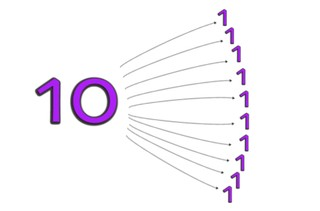Solving the division table of 10

# Solving the division table of 10

Students learn to solve the division table of 108,000 schools use Gynzy92,000 teachers use Gynzy1,600,000 students use Gynzy

## General

The students learn to solve the division table of 10.

## Standards

CCSS.Math.Content.3.OA.B.5/6

## Learning objective

Students will be able to solve the division table of 10.

## Introduction

You ask student volunteers to calculate the jumps on the number line. You do this both forwards and backwards.

## Instruction

First you discuss the importance of calculating division problems. Next you explain that in a division problem you have a certain amount in each group and that in the division table of 10 you are dividing by 10 each time. For this you show an example of 20 dollars that you divide evenly between 10 hands. From here you can use the bar on the bottom right to navigate through the lesson (visual, abstract, story) in the order that best matches the learning goals of your classroom. Otherwise you continue following the pages of the lesson in order and move on to problems with visual support. Here you explain how you solve a division problem by telling how a division problem works with images of chocolates that have to be divided between 10 hands, next you practice by dividing carrots between 10 rabbits. After this you show images and the students must come up with the problem that describes each one. Next you have the students solve division problems on their own using an image. Then you discuss abstract problems. First you show what dividing by 10 looks like when you divide the number 10 evenly. After this you show the problems of the division table of 10 and show that the division table of 10 is the same as the division table of 1 but with a 0 at the end, and that you can solve division problems with 10 by flipping the numbers to make a multiplication problem. Next the students practice using these insights. Then you walk the students through the steps of solving a story problem. The students can click on the speakers to hear each story.

Check whether the students can solve the division table of 10 by asking them the following questions:
- What does the problem 20 ÷ 10 mean? Can you name an example?
- What trick can you use to solve the division table of 10?

## Quiz

The students test their understanding of solving the division table of 10 through ten exercises. Some of these exercises include visual support, others are abstract problems and other exercises are story problems.

## Closing

You have the students solve another problem with visual support, then they solve another abstract problem and then one more story problem. To close you can play a game of tag. A student says the name of another student and a problem from the division table of 10. The student who was just named must give the answer before they are tagged, otherwise they become "it".

## Teaching tips

You can have the students use concrete materials to divide into 10 groups.

## Instruction materials

MAB blocks or other concrete materials for each pair of students

### The online teaching platform for interactive whiteboards and displays in schools

• Save time building lessons

• Manage the classroom more efficiently

• Increase student engagement